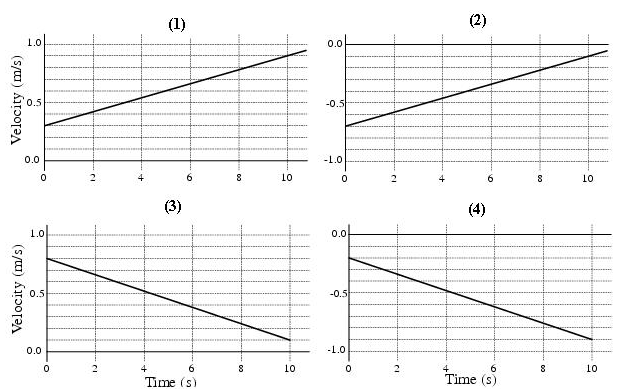# Problem: Which graphs represent an object that has a negative acceleration? A. 1 and 4 B. 1 and 3 C. 2 and 3 D. 1 and 2 E. 2 and 4 F. 3 and 4

###### FREE Expert Solution

The slope of a velocity-time graph is the acceleration.###### Problem Details

Which graphs represent an object that has a negative acceleration?A. 1 and 4

B. 1 and 3

C. 2 and 3

D. 1 and 2

E. 2 and 4

F. 3 and 4